# Rain Cloud Plots using Half Violin Plot with jittered data points in R

• Last Updated : 28 Nov, 2021

In this article, we will discuss how to create Rain Cloud Plots using Half Violin Plot with jittered data points in R

Raincloud plots or Half Violin plots are basically used to visualize the distribution and the overall summary of the data at the same time. This plot is a combination of half violin plots with jittered points on top, boxplots and can be further enhanced by adding central measures of tendency, quartile ranges, etc.

## Dataset Used

Here let’s use the “iris” dataset. This is an in-built dataset in R.

## R

 `# Loading the packages  ` `library``(ggplot2) ` `library``(plyr) ` ` `  `# load the iris data ` `df <- iris  ` `head``(df) `

Output: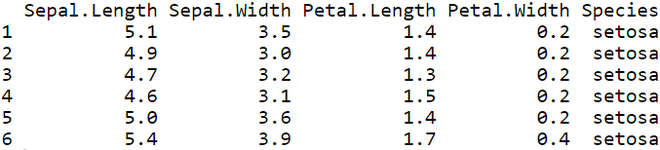## Plotting a Raincloud using ggplot

Example 1: Simple Raincloud plot

Let’s plot a Raincloud/Half Violin plot for Species vs Sepal Length of iris dataset.

## R

 `# Loading the packages  ` `library``(``"ggplot2"``) ` `library``(``"plyr"``) ` ` `  `# load the iris data ` `df <- iris  ` ` `  `# Code to plot a raincloud ` `ggplot``(df, ``aes``(Species, Sepal.Length, fill=Species)) + ` `geom_flat_violin``() + ` `coord_flip``() + ` `theme``(legend.position = ``"none"``) `

Output: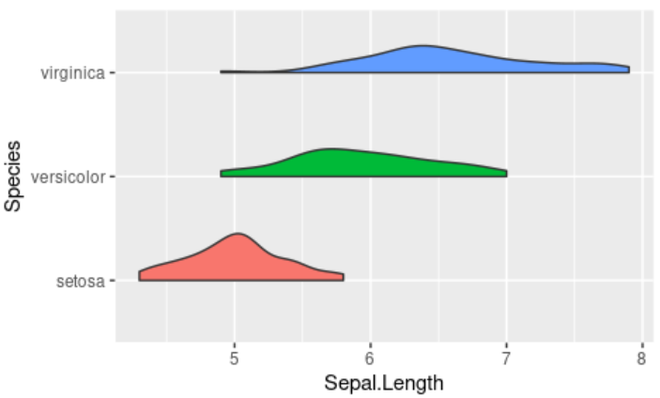Example 2: Raincloud with jittered data points on top

Let’s check out how to add jittered data points on top of the Raincloud plot using the geom_jitter( ) function.

## R

 `# Loading the packages  ` `library``(``"ggplot2"``) ` `library``(``"plyr"``) ` ` `  `# load the iris data ` `df <- iris  ` ` `  `# Code for Raincloud plot with jittered data points ` `ggplot``(df, ``aes``(Species, Sepal.Length, fill=Species)) + ` `geom_flat_violin``() + ` `coord_flip``() + ``geom_jitter``(alpha = 0.5,width = 0.15)+ ` `theme``(legend.position = ``"none"``) `

Output: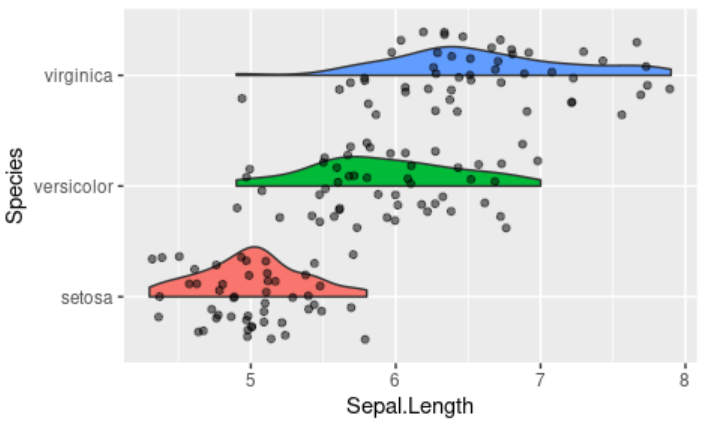Example 3: Positioning the Raincloud plot

Let’s see how to adjust the position of the Half violin plots with respect to the jittered data points.

## R

 `# Loading the packages ` `library``(``"ggplot2"``) ` `library``(``"plyr"``) ` ` `  `# load the iris data ` `df < - iris ` ` `  `# Code for positioning the raincloud plot ` `ggplot``(df, ``aes``(Species, Sepal.Length, fill=Species)) + ` `geom_flat_violin``(position=``position_nudge``(x=.2, y=0)) + ` `coord_flip``() + ``geom_jitter``(alpha=0.5, width=0.15, ``aes``(color=Species)) + ` `theme``(legend.position=``"none"``) `

Output: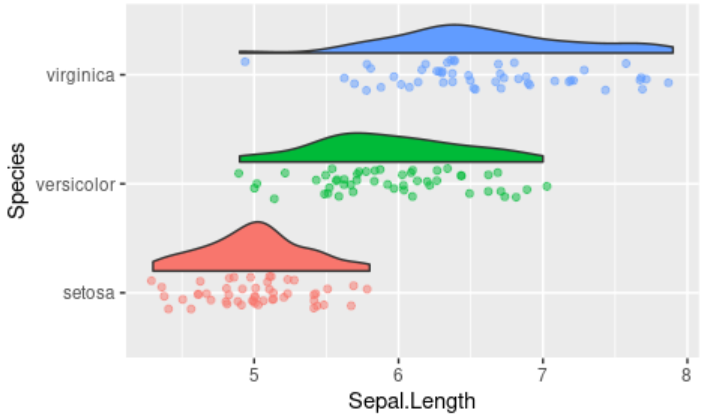Example 4: Raincloud Plot with Boxplots

Let’s see how to plot a Boxplot alongside the half violin plot in order to get additional insights of the features.

## R

 `# Loading the packages ` `library``(``"ggplot2"``) ` `library``(``"plyr"``) ` ` `  `# load the iris data ` `df < - iris ` ` `  `# Code to plot Raincloud and boxplot  ` `ggplot``(df, ``aes``(Species, Sepal.Length, fill=Species)) + ` `geom_flat_violin``(position = ``position_nudge``(x = .2, y = 0)) + ` `coord_flip``() + ``geom_jitter``(alpha = 0.5,width = 0.15) + ` `theme``(legend.position = ``"none"``) + ` `geom_boxplot``( width = .25,  outlier.shape = ``NA` `)  `

Output: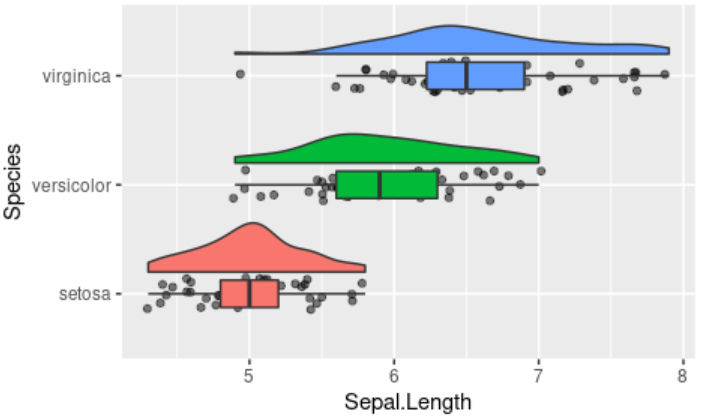My Personal Notes arrow_drop_up
Recommended Articles
Page :